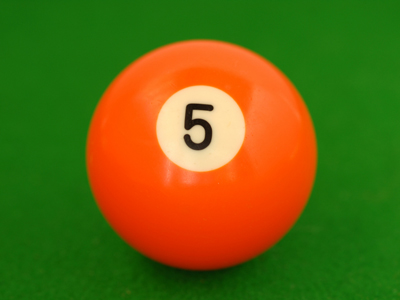To divide by 5, first multiply by 2 and then move the digits to the right.

# Multiplication and Division 2 (Medium)

This is the second of our medium level Eleven Plus maths quizzes on multiplication and division. In the first, we learned some handy techniques for multiplying and dividing which, if you remember them, will improve your mental arithmetic considerably. The best way to remember something of course, is to practice!

• To multiply by 11, first multiply by 10, then add the original number
• To multiply by 9, first multiply by 10, then subtract the original number
• To divide by 5, first multiply by 2 and then move the digits to the right
• To divide by 25, divide by 100, then multiply by 4

There are many more useful strategies you can use – you may even have some of your own. Whatever method you choose, as long as you reach the correct answer quickly, then it’s fine. Now try these teasers and see how you fare. Good luck!

1.
What is the correct answer to the given calculation?
627 × 25 = ?
15,675
15,765
15,576
15,756
To multiply a number by 25: multiply by 100, then divide by 4:
627 × 25 = (627 x 100) ÷ 4 = 62,700 ÷ 4 = 15,675
2.
What is the correct answer to the given calculation?
212 × 9 = ?
1,809
1,098
1,980
1,908
To multiply a number by 9: first multiply the number by 10, then subtract the original number from it:
212 × 9 = (212 x 10) - 212 = 2,120 - 212 = 1,908
3.
What is the correct answer to the given calculation?
134 × 12 = ?
1,608
1,806
1,068
1,680
To multiply a number by 12, first multiply the number by 10, then add twice the original number to it:
134 × 12 = (134 x 10) + (134 x 2) = 1,340 + 268 = 1,608
4.
What is the correct answer to the given calculation?
96 × 80 = ?
7,608
7,860
7,086
7,680
To multiply a number by 80, multiply the number by 2, then by 2 again, and again by 2, and finally by 10:
96 × 80 = (96 x 2 x 2 x 2) x 10 = (192 x 2 x 2) x 10 = (384 x 2) x 10 = 768 x 10 = 7,680
5.
What is the correct answer to the given calculation?
27 × 101 = ?
27,270
272.7
2,727
2,277
To multiply a number by 101, first multiply by 100 then add the original number:
27 × 101 = (27 x 100) + 27 = 2,700 + 27 = 2,727
6.
What is the correct answer to the given calculation?
475 ÷ 25 = ?
17
18
19
20
To divide a number by 25: divide by 100, then multiply by 4:
475 ÷ 25 = (475 ÷ 100) x 4 = 4.75 x 4 = 19
7.
What is the correct answer to the given calculation?
326 ÷ 5 = ?
0.652
652
6.52
65.2
To divide a number by 5: first multiply by 2 and then move the digits to the right:
326 ÷ 5 = (326 × 2) ÷ 10 = 652 ÷ 10 . Move the d.p. 1 place to the left gives 65.2
8.
What is the correct answer to the given calculation?
714 × 11 = ?
7,548
7,485
7,854
7,845
To multiply a number by 11, first multiply the number by 10, then add the original number to it:
714 x 11 = (714 x 10) + 714 = 7,140 + 714 = 7,854
9.
What is the correct answer to the given calculation?
394,227 ÷ 1,000 = ?
3.94227
394.227
3,942.27
39.4227
To divide a number by a multiple of 10 whose only non-zero digit is the leading digit 1: move the digits to the right by the same number of zeros that are in the multiple of 10, in this case 3 places as there are 3 zeros in 1,000
10.
What is the correct answer to the given calculation?
2,343 × 5 = ?
11,157
11,715
11,571
11,175
To multiply a number by 5: first divide by 2, then move the digits to the left:
2,343 × 5 = (2,343 ÷ 2) x 10 = 1,171.5 x 10. Move the d.p. 1 place to the right gives 11,715
Author:  Frank Evans